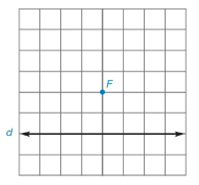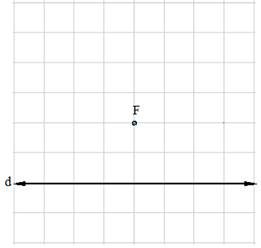Chapter 7.1, Problem 23EElementary Geometry For College St...

7th Edition
Alexander + 2 others
ISBN: 9781337614085

Solutions

Chapter
SectionElementary Geometry For College St...

7th Edition
Alexander + 2 others
ISBN: 9781337614085
Textbook Problem

Note: Exercises preceded by an asterisk are of a more challenging nature.For Exercises 23 to 28, use the grid and your compass (as needed) to locate several points on the parabola having the given focus F and directrix d . Then sketch the parabola that is characterized by these points.To determine

To sketch:

The parabola using the below given grid with directrix and focus.Explanation

Definition:

A parabola is the locus of points that are equidistant from a fixed line and a fixed point not on that line.

The fixed point is known as focus of the parabola and the fixed line is known as directrix of the parabola.

Procedure:

1. Draw the given directrix d and focus F and locate a point D1.

2. Locate a point P1 which is the mid-point of FD1. The point P1 will be the vertex.

3. Locate the points P1, P2, P3, P4, and P5 such that D1P1=P1F, D2

Still sussing out bartleby?

Check out a sample textbook solution.

See a sample solution

The Solution to Your Study Problems

Bartleby provides explanations to thousands of textbook problems written by our experts, many with advanced degrees!

Get Started

Use the Midpoint Rule with n = 6 to approximate 03sin(x3)dx.

Single Variable Calculus: Early Transcendentals, Volume I

let f(x) = x3 + 5, g(x) = x2 2, and h(x)= 2x + 4, Find the rule for each function. 1. f + g

Applied Calculus for the Managerial, Life, and Social Sciences: A Brief Approach

In Exercises 1-4, simplify the expression. x25x6x29x+18

Calculus: An Applied Approach (MindTap Course List)

Expand each expression in Exercises 122. (3x+1)(2x2x+1)

Finite Mathematics and Applied Calculus (MindTap Course List)